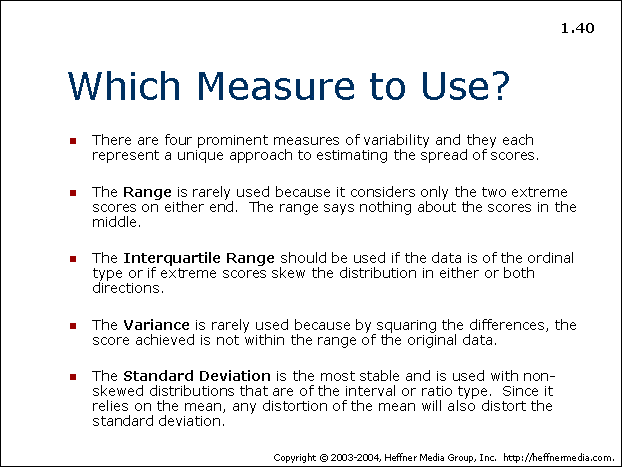# 40: Measures: Range, Variance, Standard Deviation## Which Measure to Use?

• There are four prominent measures of variability and they each represent a unique approach to estimating the spread of scores.
• The Range is rarely used because it considers only the two extreme scores on either end. The range says nothing about the scores in the middle.
• The Interquartile Range should be used if the data is of the ordinal type or if extreme scores skew the distribution in either or both directions.
• The Variance is rarely used because by squaring the differences, the score achieved is not within the range of the original data.
• The Standard Deviation is the most stable and is used with non-skewed distributions that are of the interval or ratio type. Since it relies on the mean, any distortion of the mean will also distort the standard deviation.